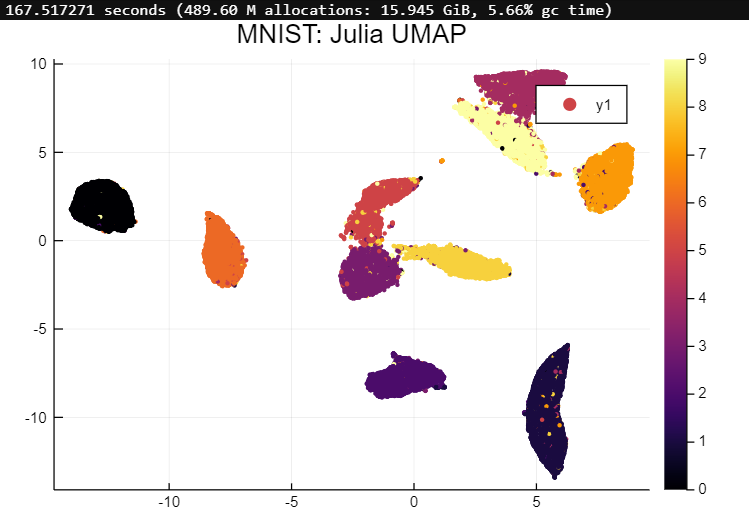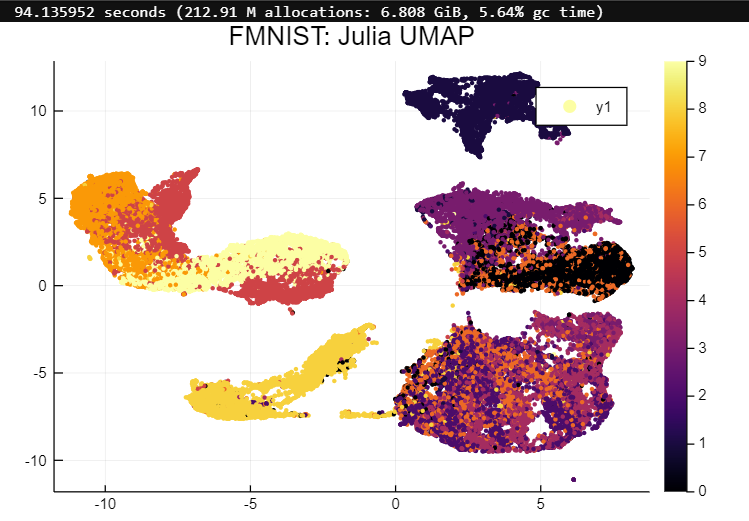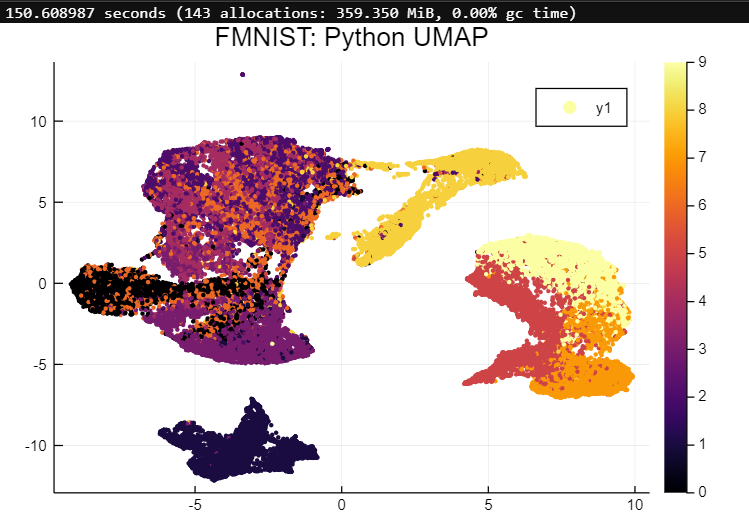78

10

10

6

# UMAP.jl

A pure Julia implementation of the Uniform Manifold Approximation and Projection dimension reduction algorithm

McInnes, L, Healy, J, Melville, J, UMAP: Uniform Manifold Approximation and Projection for Dimension Reduction. ArXiV 1802.03426, 2018

## Usage

``````embedding = umap(X, n_components; n_neighbors, metric, min_dist, ...)
``````

The `umap` function takes two arguments, `X` (a column-major matrix of shape (n_features, n_samples)), `n_components` (the number of dimensions in the output embedding), and various keyword arguments. Several important ones are:

• `n_neighbors::Int=15`: This controls how many neighbors around each point are considered to be part of its local neighborhood. Larger values will result in embeddings that capture more global structure, while smaller values will preserve more local structures.
• `metric::SemiMetric=Euclidean()`: The (semi)metric to use when calculating distances between points. This can be any subtype of the `SemiMetric` type from the `Distances.jl` package, including user-defined types.
• `min_dist::Float=0.1`: This controls the minimum spacing of points in the embedding. Larger values will cause points to be more evenly distributed, while smaller values will preserve more local structure.

The returned `embedding` will be a matrix of shape (n_components, n_samples).

### Using precomputed distances

UMAP can use a precomputed distance matrix instead of finding the nearest neighbors itself. In this case, the distance matrix is passed as `X` and the `metric` keyword argument should be `:precomputed`. Example:

``````embedding = umap(distances, n_components; metric=:precomputed)
``````

## Fitting a UMAP model to a dataset and transforming new data

### Constructing a model

To construct a model to use for embedding new data, use the constructor:

``````model = UMAP_(X, n_components; )
``````

where the constructor takes the same keyword arguments (kwargs) as `umap`. The returned object has the following fields:

``````model.graph     # The graph of fuzzy simplicial set membership strengths of each point in the dataset
model.embedding # The embedding of the dataset
model.data      # A reference to the original dataset
model.knns      # A matrix of indices of nearest neighbors of points in the dataset,
# as determined on the original manifold (may be approximate)
model.dists     # The distances of the neighbors indicated by model.knns
``````

### Embedding new data

To transform new data into the existing embedding of a UMAP model, use the `transform` function:

``````Q_embedding = transform(model, Q; )
``````

where `Q` is a matrix of new query data to embed into the existing embedding, and `model` is the object obtained from the `UMAP_` call above. `Q` must come from a space of the same dimensionality as `model.data` (ie `X` in the `UMAP_` call above).

The remaining keyword arguments (kwargs) are the same as for above functions.

## Implementation Details

There are two main steps involved in UMAP: building a weighted graph with edges connecting points to their nearest neighbors, and optimizing the low-dimensional embedding of that graph. The first step is accomplished either by an exact kNN search (for datasets with `< 4096` points) or by the approximate kNN search algorithm, NNDescent. This step is also usually the most costly.

The low-dimensional embedding is initialized (by default) with the eigenvectors of the normalized Laplacian of the kNN graph. These are found using ARPACK (via Arpack.jl).

## Current Limitations

• Input data types: Only data points that are represented by vectors of numbers (passed in as a matrix) are valid inputs. This is mostly due to a lack of support for other formats in NNDescent. Support for e.g. string datasets is possible in the future
• Sequential: This implementation does not take advantage of any parallelism

## External Resources

• Understanding UMAP
• For a great description of how UMAP works, see this page from the Python UMAP documentation
• If you're familiar with t-SNE, then this page describes UMAP with similar vocabulary to that dimension reduction algorithm

## Examples

The full MNIST and FMNIST datasets are plotted below using both this implementation and the Python implementation for comparison. These were generated by this notebook.

Note that the memory allocation for the Python UMAP is unreliable, as Julia's benchmarking doesn't count memory allocated within Python itself.

### MNIST### FMNIST## Disclaimer

This implementation is a work-in-progress. If you encounter any issues, please create an issue or make a pull request.

09/13/2018